Latest Banking jobs   »   Quantitative Aptitude Quiz For IBPS Clerk...

# Quantitative Aptitude Quiz For IBPS Clerk Prelims 2021- 23rd August

Directions (1 – 5): In each of these questions, two equations (I) and (II) are given. You have to solve both the equations and answer the following questions.
(a) x > y
(b) x < y
(c) x ≥ y
(d) x ≤ y
(e) x = y or no relation.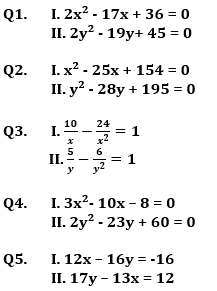Directions (6-10): In each of these questions, two equations (I) and (II) are given. You have to solve both the equations and give answer
(a) if x>y
(b) if x≥y
(c) if x<y
(d) if x ≤y
(e) if x = y or no relation can be established between x and y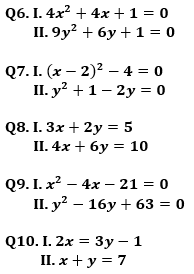Directions (11-15): – In each of these questions, two equations (I) and (II) are given. You have to solve both the equations and give answer
(a) if x>y
(b) if x≥y
(c) if x<y
(d) if x ≤y
(e) if x = y or no relation can be established between x and y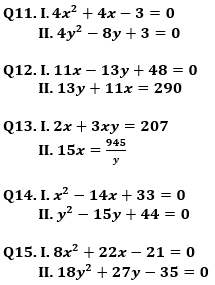Solutions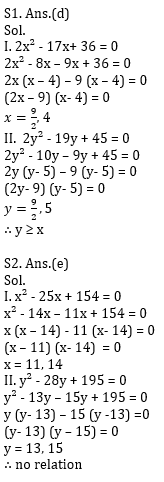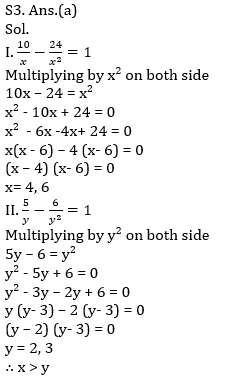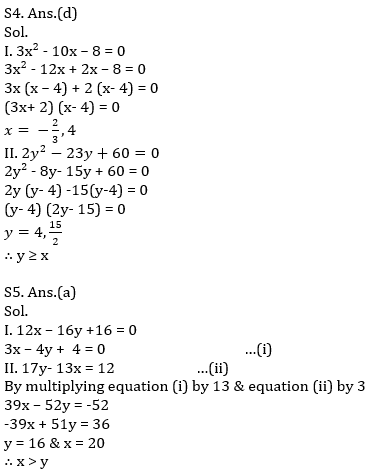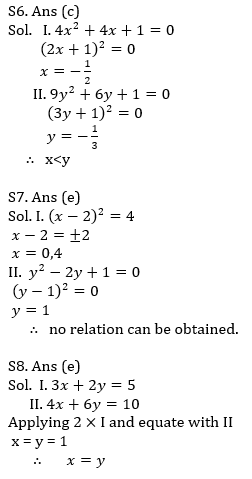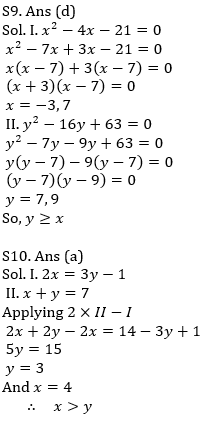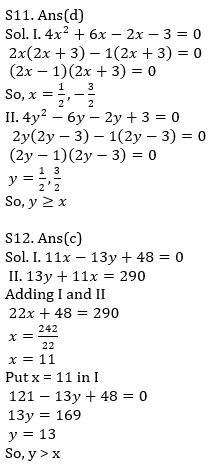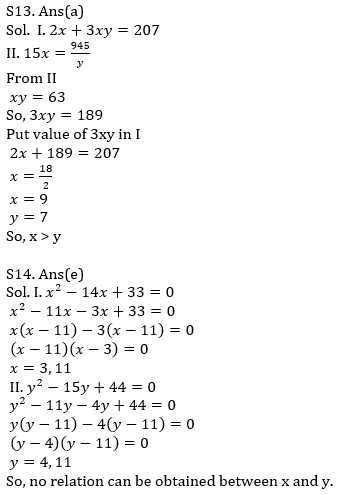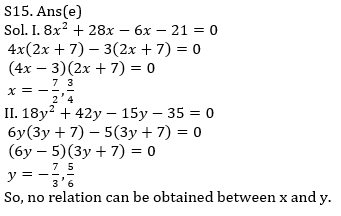#### Congratulations!Incorrect details? Fill the form again here

•Reasoning Ability Quiz For IBPS Clerk Pr...
•Quantitative Aptitude Quiz For IBPS Cler...
•English Quizzes For IBPS Clerk Prelims 2...
•Quantitative Aptitude Quiz For IDBI AM/E...
•Quantitative Aptitude Quiz For IDBI AM/E...
•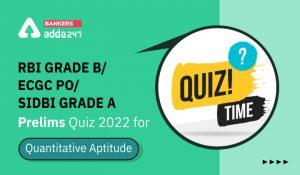Quantitative Aptitude Quiz For RBI Grade...